GeeksforGeeks App
Open AppBrowser
Continue

# Plotting graphs using Python’s plotly and cufflinks module

plotly is a Python library which is used to design graphs, especially interactive graphs. It can plot various graphs and charts like histogram, barplot, boxplot, spreadplot and many more. It is mainly used in data analysis as well as financial analysis. plotly is an interactive visualization library.

cufflink connects plotly with pandas to create graphs and charts of dataframes directly. choropleth is used to describe geographical plotting of USA. choropleth is used in the plotting of world maps and many more.

Let’s plot different types of plots like boxplot, spreadplot, etc. using plotly and cufflinks.

Command to install plotly:

`pip install plotly `

`pip install cufflink`

Code #1: Show dataframe

 `# import all necessary libraries``import` `pandas as pd``import` `numpy as np`` ` `%` `matplotlib inline``from` `plotly ``import` `__version__``import` `cufflinks as cf`` ` `from` `plotly.offline ``import` `download_plotlyjs, init_notebook_mode, plot, iplot`` ` `# to get the connection``init_notebook_mode(connected ``=` `True``)`` ` `# plotly also serves online,``# but we are using just a sample``cf.go_offline()`` ` `# creating dataframes``df ``=` `pd.DataFrame(np.random.randn(``100``, ``4``), columns ``=``'A B C D'``.split())`` ` `df2 ``=` `pd.DataFrame({``'Category'``:[``'A'``, ``'B'``, ``'C'``], ``'Values'``:[``32``, ``43``, ``50``]})``df2.head()`

Output: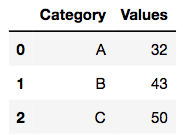Code #2: Normal Plot

 `# plotly function``df.iplot()`

Output: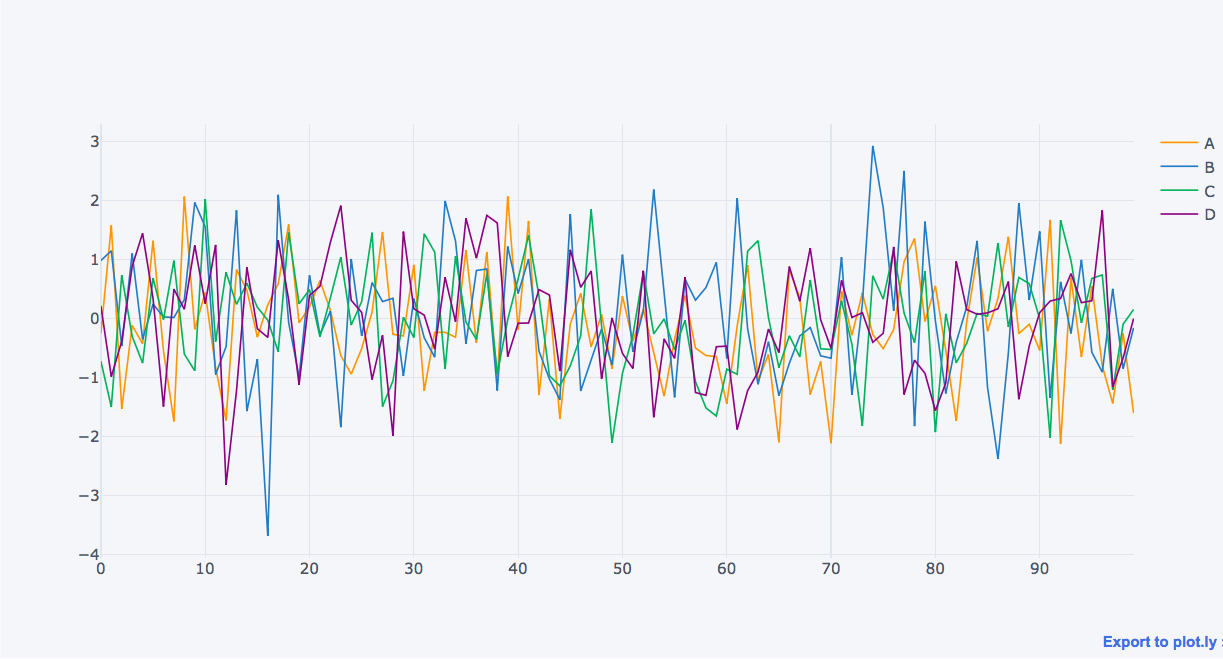Code #3: Scatter Plot

 `# markers are made to point in the graph``df.iplot(kind ``=``'scatter'``, x ``=``'A'``, y ``=``'B'``, mode ``=``'markers'``)`

Output: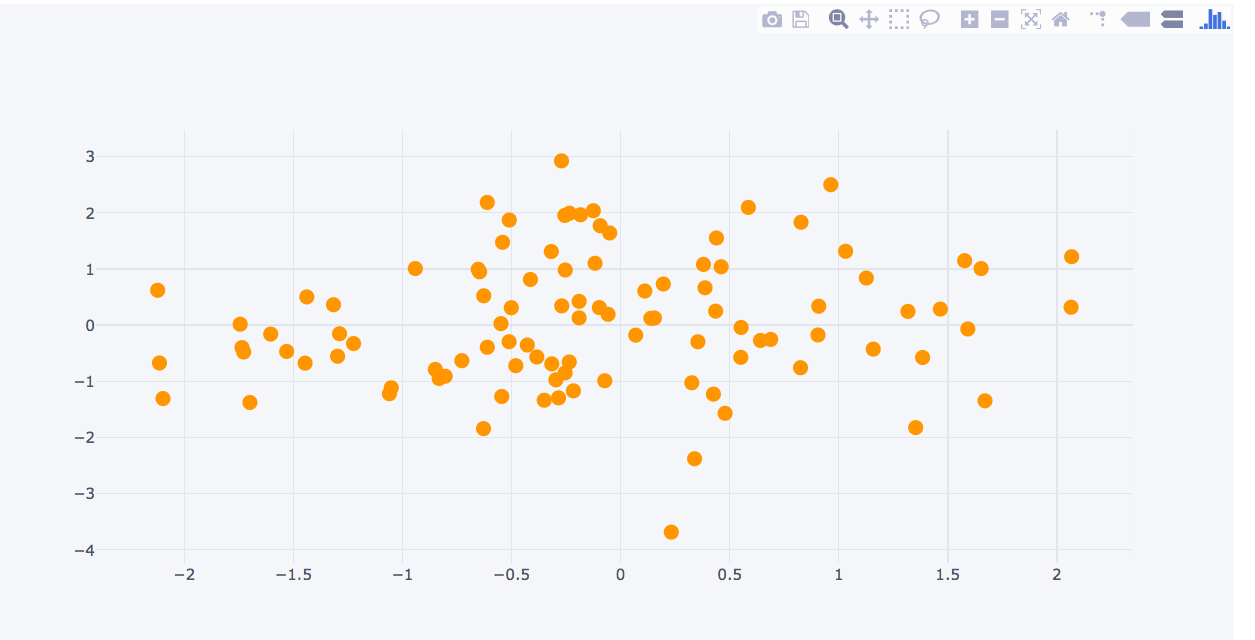Code #4: Box Plot

 `# boxplot``df.iplot(kind ``=``'box'``)`

Output: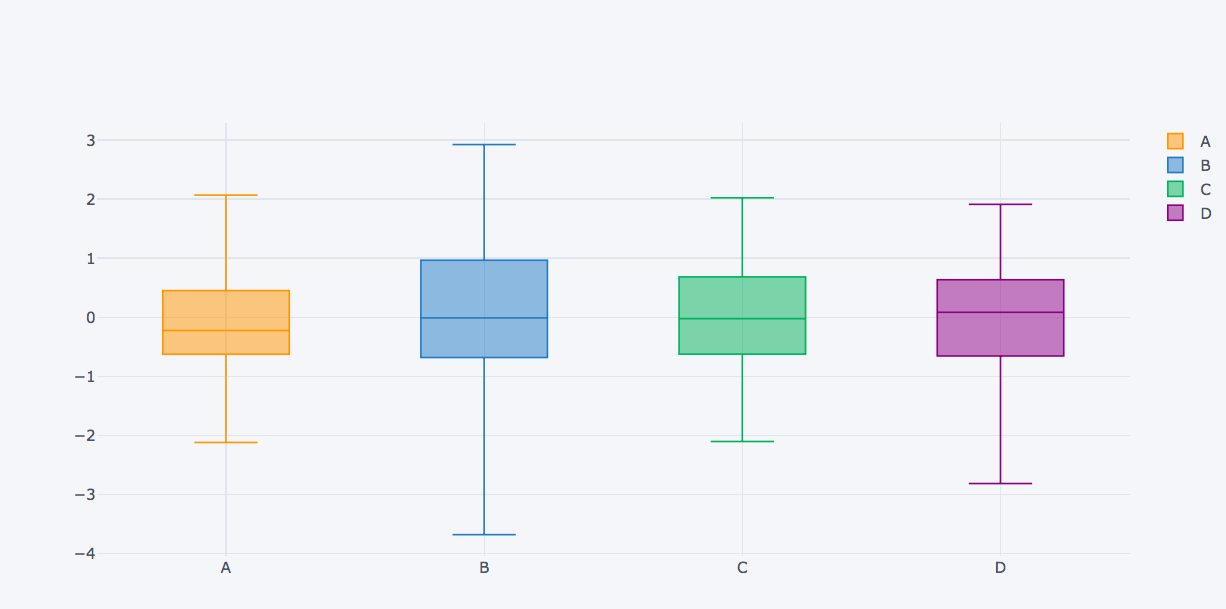Code #5: Plot dataframes

 `# creating dataframe with three axes``df3 ``=` `pd.DataFrame({``'x'``:[``1``, ``2``, ``3``, ``4``, ``5``],``                    ``'y'``:[``10``, ``20``, ``30``, ``20``, ``10``],``                    ``'z'``:[``5``, ``4``, ``3``, ``2``, ``1``]})`

Output: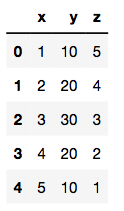Code #6: Surface plot

 `# surface plot``# colorscale:red(rd), yellow(yl), blue(bu)``df3.iplot(kind ``=``'surface'``, colorscale ``=``'rdylbu'``)`

Output: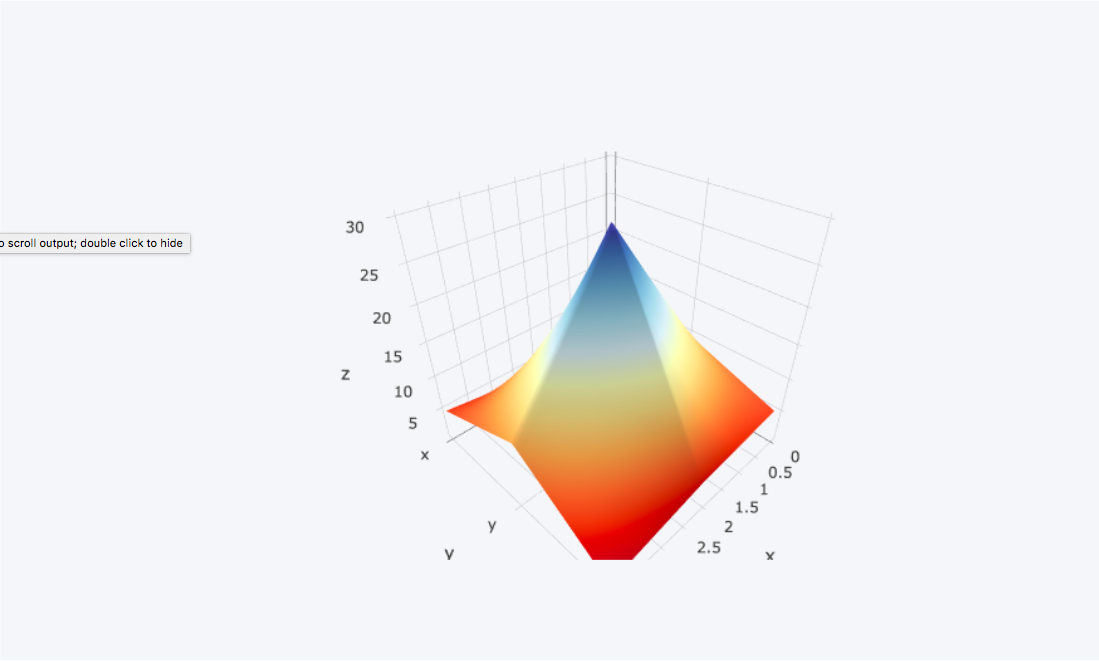My Personal Notes arrow_drop_up